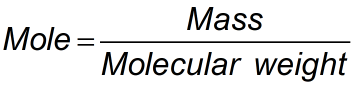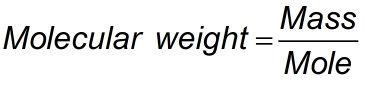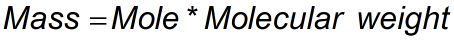Request a Tool

Mole Calculator

Mole is an SI unit for the amount of a substance.

Moles (mol)
0

Molecular Weight
0

Mass M
0

FormulaDefination / Uses

The mole is the International System of Units' fundamental unit of material quantity. It is defined as the collection of exactly 6.022140761023 particles, that might be atoms, molecules, ions, or electrons.

FormulaDefination / Uses

The mole is the International System of Units' fundamental unit of material quantity. It is defined as the collection of exactly 6.022140761023 particles, that might be atoms, molecules, ions, or electrons.

FormulaDefination / Uses

The mole is the International System of Units' fundamental unit of material quantity. It is defined as the collection of exactly 6.022140761023 particles, that might be atoms, molecules, ions, or electrons.

The mole is a unit of measurement for quantity. one mole of substance has the same amount of atoms, molecules, and other particles. Our Mole Calculator will help you to determine the mole of a substance. All you have to do is enter the mass and molecular weight of the substance. As of May 20, 2019, a mole is defined as the amount of a chemical substance that contains exactly 6.022140761023 (Avogadro's constant) atoms, molecules, ions, or electrons (constitutive particles) according to the official International System of Units definition. A mole was previously defined as the number of atoms contained in 12 grammes of carbon-12 (an isotope of carbon). Use the upper given formula for manual calculations. No sign-up, registration OR captcha is required to use this tool.